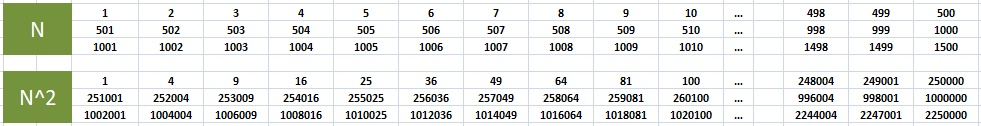Patterns in Powers of Natural Numbers

Page 1 of 2

Every discovery begins with a question. The inquiry in this case was - "If we know or memorize squares of a few numbers, say 1-25, is it possible to quickly calculate squares of larger numbers?". The search for an answer pointed to finding out how last n digits of squares, and higher powers, form a repeating sequence. Specfically, it leads us to - "Squares of natural numbers always have the last digit as 0,1,4,5,6, or 9. Is there a similar restriction on last 2 digits? Last n digits? Do these trailing digits form a pattern? How about higher powers of natural numbers?".

First, let's make a small observation about square numbers. Square numbers always end in one of 0,1,4,5,6,9.
These trailing digits repeat over and over again. Do they form any pattern? Yes!
To reveal the pattern, natural numbers are arranged in an array of 1-10, 11-20, so on, and their squares listed in another array. We find that the last digit of squares of 1-10, is same as last digit of squares of 11-20, etc.The next natural (pun intended) question is, whether a similar pattern exists for last 2 trailing digits.
Using basic MS Excel features, we find that in this case, the pattern length is 50.By now, the reader has guessed that a similar pattern exists for last 3 digits of squares as well. Before we go there, let's take a moment to make another observation. The sequence of length 50 that we just saw, also has a "sub-pattern" of length 25. To see this, we arrange the squares of 1-50 in the following order.Now we notice that squares of 26 and 24 differ by 100, of 27 and 23 differ by 200, and so on.
If we know the squares of numbers from 1-25, knowing this pattern makes it easy to find squares of next 25 numbers.

For example: square of 44? We see that 44 and 6 (i.e 50-44) share same last 2 digits, and they differ by (25-6)*100 = 1900.
Thus 44 square is 1936.

There are other ways to find squares of numbers closer to 25, 50, and 100. We leave this for reader to research on.

The sub-pattern does not end at 100. It carries on for rest of the natural numbers, and can be observed if we continue the zig zag way of forming the number array. That is, writing 51 on the next line below 49, up to 74, and then writing 76 below 74 on a new line and continuing to the left, and then towards right on the next line, and so on.
However, the difference between 2 successive lines is no longer limited to 100, and continues to increase.

Returning to our earlier discussion, we can see from the table below that the pattern for last 3 digits of squares repeats every 500 numbers.For conversation sake, let's call the earliest point where the sequence resets as "Reset point" or "Tipping point".
Do the higher powers exhibit similar pattern? If so, can we predict the tipping points?
Let's continue the discussion on Page 2.

 Quick Comment : * Name Email ( Your email id is safe with us! ) * Comments * Type the number of characters in the word "HUMAN"